Normal view

# Introduction to Bayesian statistics / William M. Bolstad.

Material type:TextLanguage: English Publication details: c2007. Edition: 2nd edDescription: xxiv, 437 p. : ill. ; 25 cmISBN: 9780470141151; 0470141158Subject(s): Bayesian statistical decision theoryDDC classification: 519.542 LOC classification: QA279.5 | .B65 2007
Contents:
TOC Introduction to statistical science -- Scientific data gathering -- Displaying and summarizing data -- Logic, probability, and uncertainty -- Discrete random variables -- Bayesian inference for discrete random variables -- Continuous random variables -- Bayesian inference for binomial proportion -- Comparing Bayesian and frequentist inferences for proportion -- Bayesian inference for poisson -- Bayesian inference for normal mean -- Comparing Bayesian and frequentist inferences for mean -- Bayesian inference for difference between means -- Bayesian inference for single linear regression -- Bayesian inference for standard deviation -- Robust Bayesian methods.
Summary: Summary: Covers the topics typically found in an introductory statistics book-but from a Bayesian perspective-giving readers an advantage as they enter fields where statistics is used.
Tags from this library: No tags from this library for this title.
Star ratingsAverage rating: 0.0 (0 votes)
Holdings
Item type Current library Collection Call number Copy number Status Date due Barcode Item holdsE-Book
E-book
Non-fiction 519.542 BOI 2007 (Browse shelf(Opens below)) Not For LoanText
Reserve Section
Non-fiction 519.542 BOI 2007 (Browse shelf(Opens below)) C-1 Not For Loan 25697
Total holds: 0
##### Browsing EWU Library shelves, Shelving location: E-book Close shelf browser (Hides shelf browser)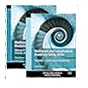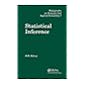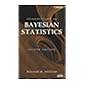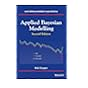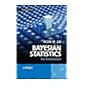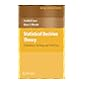519.54 RAM 2012 Multilevel and longitudinal modeling using Stata / 519.54 SIS 2003 Statistical inference / 519.54 SPA 2007 Applied nonparametric statistical methods / 519.542 BOI 2007 Introduction to Bayesian statistics / 519.542 COA 2014 Applied Bayesian modelling / 519.542 LEB 2012 Bayesian statistics : 519.542 LIS 2008 Statistical decision theory :

Online version:
Introduction to Bayesian statistics.
Hoboken, N.J. : John Wiley, c2007
(OCoLC)654593316

Includes bibliographical references (p. 431-432) and index.

TOC Introduction to statistical science --
Scientific data gathering --
Displaying and summarizing data --
Logic, probability, and uncertainty --
Discrete random variables --
Bayesian inference for discrete random variables --
Continuous random variables --
Bayesian inference for binomial proportion --
Comparing Bayesian and frequentist inferences for proportion --
Bayesian inference for poisson --
Bayesian inference for normal mean --
Comparing Bayesian and frequentist inferences for mean --
Bayesian inference for difference between means --
Bayesian inference for single linear regression --
Bayesian inference for standard deviation --
Robust Bayesian methods.

Summary:
Covers the topics typically found in an introductory statistics book-but from a Bayesian perspective-giving readers an advantage as they enter fields where statistics is used.

AS

Tahur Ahmed

There are no comments on this title.